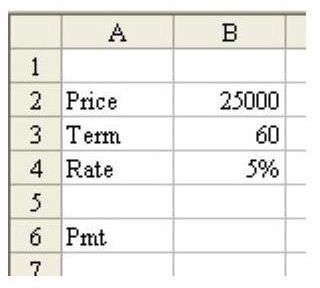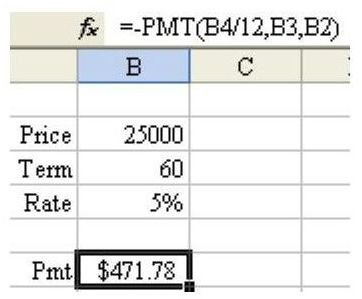# How to Calculate a Loan Payment in Excel

## Excel and Loan PaymentsProblem: You are considering buying a car. You want to calculate the loan payment in Excel, as shown in Fig. 325. (Click any image for a larger view.)

Not only can the method described in the next section let you calculate the loan payment for one item, but once the formula has been entered, you can change the values for Price, Term, and Rate to see how making minor adjustments to these items will affect the monthly loan payment. For example, you could change the term from 5 years (60 months) to 3 years (36 months) to see if you could afford to pay the car off faster and save yourself from added interest charges.

## How to Calculate a Loan Payment

Strategy: Use the PMT function. Follow these steps.

1) Enter Price, Term in Months, and Annual Percentage Rate in cells B2:B4, as shown in Fig. 325 in the previous section.

The PMT function has three required arguments. The first argument is the interest rate. The second argument is the number of payments in the loan. The final argument is the original loan amount.

Gotcha: The interest rate must be entered as a percentage. If you are planning on monthly payments (which is normal), then you have to divide the annual percentage rate by twelve.

Gotcha: In financial terms, the bank is loaning you \$25,000 – a positive amount coming to you. Thus, the payments that you make to the bank are really a negative amount – it is money leaving your wallet. For this reason, the result of the PMT function will be negative. However, you can precede the PMT function with a minus sign in order to return a positive payment amount.

2) Enter this formula in cell B6: =–PMT(B4/12,B3,B2). See Fig. 326.Summary: The PMT function is great at calculating house or car loans.

Functions Discussed: =PMT()

## More Excel Tips

For more Excel tips and tutorials, please be sure to check out the hundreds of other resources available at Bright Hub. In particular, you may be interested in the following collections.

91 Tips for Calculating with Microsoft Excel – This set of 91 tutorials focuses on shortcuts and strategies that can be used to get the most out of Excel’s high-powered calculation tools.

Excel Formatting Tips from Mr. Excel – Taken from the book Learn Excel from Mr. Excel, these 72 Excel formatting tips offer advice on how to better organize, analyze, and present your data.

How to Use Functions in Microsoft Excel – The functions in Excel do more than just calculate numerical values. They can also be used to look up information in a table, manipulate text, and more. Find out more about the varied uses of Excel functions in this set of tutorials.